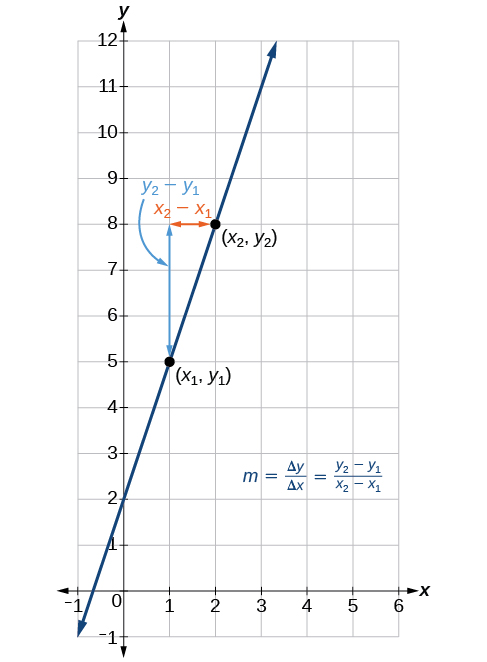# 4.1 Linear functions  (Page 3/27)

 Page 3 / 27

## Increasing and decreasing functions

The slope determines if the function is an increasing linear function    , a decreasing linear function    , or a constant function.

• $f\left(x\right)=mx+b\text{\hspace{0.17em}}$ is an increasing function if $\text{\hspace{0.17em}}m>0.$
• $f\left(x\right)=mx+b\text{\hspace{0.17em}}$ is a decreasing function if $\text{\hspace{0.17em}}m<0.$
• $f\left(x\right)=mx+b\text{\hspace{0.17em}}$ is a constant function if $\text{\hspace{0.17em}}m=0.$

## Deciding whether a function is increasing, decreasing, or constant

Some recent studies suggest that a teenager sends an average of 60 texts per day http://www.cbsnews.com/8301-501465_162-57400228-501465/teens-are-sending-60-texts-a-day-study-says/ . For each of the following scenarios, find the linear function that describes the relationship between the input value and the output value. Then, determine whether the graph of the function is increasing, decreasing, or constant.

1. The total number of texts a teen sends is considered a function of time in days. The input is the number of days, and output is the total number of texts sent.
2. A teen has a limit of 500 texts per month in his or her data plan. The input is the number of days, and output is the total number of texts remaining for the month.
3. A teen has an unlimited number of texts in his or her data plan for a cost of \$50 per month. The input is the number of days, and output is the total cost of texting each month.

Analyze each function.

1. The function can be represented as $\text{\hspace{0.17em}}f\left(x\right)=60x\text{\hspace{0.17em}}$ where $\text{\hspace{0.17em}}x\text{\hspace{0.17em}}$ is the number of days. The slope, 60, is positive so the function is increasing. This makes sense because the total number of texts increases with each day.
2. The function can be represented as $\text{\hspace{0.17em}}f\left(x\right)=500-60x\text{\hspace{0.17em}}$ where $\text{\hspace{0.17em}}x$ is the number of days. In this case, the slope is negative so the function is decreasing. This makes sense because the number of texts remaining decreases each day and this function represents the number of texts remaining in the data plan after $\text{\hspace{0.17em}}x\text{\hspace{0.17em}}$ days.
3. The cost function can be represented as $\text{\hspace{0.17em}}f\left(x\right)=50\text{\hspace{0.17em}}$ because the number of days does not affect the total cost. The slope is 0 so the function is constant.

## Interpreting slope as a rate of change

In the examples we have seen so far, the slope was provided to us. However, we often need to calculate the slope given input and output values. Recall that given two values for the input, $\text{\hspace{0.17em}}{x}_{1}$ and $\text{\hspace{0.17em}}{x}_{2},$ and two corresponding values for the output, $\text{\hspace{0.17em}}{y}_{1}\text{\hspace{0.17em}}$ and $\text{\hspace{0.17em}}{y}_{2}\text{\hspace{0.17em}}$ —which can be represented by a set of points, and —we can calculate the slope $m.$

Note that in function notation we can obtain two corresponding values for the output $\text{\hspace{0.17em}}{y}_{1}\text{\hspace{0.17em}}$ and $\text{\hspace{0.17em}}{y}_{2}\text{\hspace{0.17em}}$ for the function $\text{\hspace{0.17em}}f,$ $\text{\hspace{0.17em}}{y}_{1}=f\left({x}_{1}\right)\text{\hspace{0.17em}}$ and $\text{\hspace{0.17em}}{y}_{2}=f\left({x}_{2}\right),$ so we could equivalently write

$m=\frac{f\left({x}_{2}\right)–f\left({x}_{1}\right)}{{x}_{2}–{x}_{1}}$

[link] indicates how the slope of the line between the points, $\text{\hspace{0.17em}}\left({x}_{1},{y}_{1}\right)\text{\hspace{0.17em}}$ and $\text{\hspace{0.17em}}\left({x}_{2},{y}_{2}\right),\text{\hspace{0.17em}}$ is calculated. Recall that the slope measures steepness, or slant. The greater the absolute value of the slope, the steeper the slant is.The slope of a function is calculated by the change in   y   divided by the change in   x .   It does not matter which coordinate is used as the   ( x 2 , y 2 )   and which is the   ( x 1 , y 1 ) ,   as long as each calculation is started with the elements from the same coordinate pair.

#### Questions & Answers

find to nearest one decimal place of centimeter the length of an arc of circle of radius length 12.5cm and subtending of centeral angle 1.6rad
factoring polynomial
find general solution of the Tanx=-1/root3,secx=2/root3
find general solution of the following equation
Nani
the value of 2 sin square 60 Cos 60
0.75
Lynne
0.75
Inkoom
when can I use sin, cos tan in a giving question
depending on the question
Nicholas
I am a carpenter and I have to cut and assemble a conventional roof line for a new home. The dimensions are: width 30'6" length 40'6". I want a 6 and 12 pitch. The roof is a full hip construction. Give me the L,W and height of rafters for the hip, hip jacks also the length of common jacks.
John
I want to learn the calculations
where can I get indices
I need matrices
Nasasira
hi
Raihany
Hi
Solomon
need help
Raihany
maybe provide us videos
Nasasira
Raihany
Hello
Cromwell
a
Amie
What do you mean by a
Cromwell
nothing. I accidentally press it
Amie
you guys know any app with matrices?
Khay
Ok
Cromwell
Solve the x? x=18+(24-3)=72
x-39=72 x=111
Suraj
Solve the formula for the indicated variable P=b+4a+2c, for b
Need help with this question please
b=-4ac-2c+P
Denisse
b=p-4a-2c
Suddhen
b= p - 4a - 2c
Snr
p=2(2a+C)+b
Suraj
b=p-2(2a+c)
Tapiwa
P=4a+b+2C
COLEMAN
b=P-4a-2c
COLEMAN
like Deadra, show me the step by step order of operation to alive for b
John
A laser rangefinder is locked on a comet approaching Earth. The distance g(x), in kilometers, of the comet after x days, for x in the interval 0 to 30 days, is given by g(x)=250,000csc(π30x). Graph g(x) on the interval [0, 35]. Evaluate g(5)  and interpret the information. What is the minimum distance between the comet and Earth? When does this occur? To which constant in the equation does this correspond? Find and discuss the meaning of any vertical asymptotes.
The sequence is {1,-1,1-1.....} has
circular region of radious
how can we solve this problem
Sin(A+B) = sinBcosA+cosBsinA
Prove it
Eseka
Eseka
hi
Joel
yah
immyByByByBy